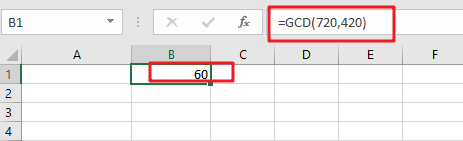# How To Use Excel GCD Function

This post will guide you how to use Excel GCD function with syntax and examples in Microsoft excel.

## Description

The Excel GCD function Returns the greatest common divisor of two or more integers. So you can use the GCD function to get the largest positive integer that divides the numbers evenly in Excel.

The GCD  function is a build-in function in Microsoft Excel and it is categorized as a MATH Function.

The GCD function is available in Excel 2016, Excel 2013, Excel 2010, Excel 2007, Excel 2011 for Mac.

## Syntax

The syntax of the GCD function is as below:

`= GCD(number1, [number2], ...)`

Where the GCD function arguments are:

• Number1 -This is a required argument. The first number.
• Number2 – This is optional argument. subsequent numbers

Note:

• If argument value is non-numeric, the GCD function will return the #VALUE! Error value.
• If argument value is less than zero, the GCD function will return the #NUM! error value.
• If one number to GCD function is greater than 2^53, the GCD function will return the #NUM! error value.

## Excel GCD Function Examples

The below examples will show you how to use Excel GCD Function to get the greatest common divisor of given numbers.

#1 to get the greatest common divisor of the numbers 720 and 420, using the following formula based on the GCD function :

`=GCD(720,420)`